﻿ Câu hỏi của Siêu Nhân Gao - Toán lớp 9 - Học toán với OnlineMath
Lỗi: Trang web OLM.VN không tải hết được tài nguyên, xem cách sửa tại đây.

# Hỏi đáp bài tập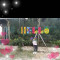Báo cáo sai phạm

4. Call that polynomial P (x) = ax3 + bx2 + cx + d

We have P(1)=a+b+c+d=3   (1)

P(2)=8a+4b+2c+d=3   (2)

P(3)=27a+9b+3c+d  =7       (3)

P(4)=64a+16b+4c+d     =21    (4)

From (1) and  (2) =>  7a+3b+c=0

From (1) and  (4)  => 63a+15b+3c=18

=> 12b+6c=-18  => 2b+c=-3

From (1) and  (3) =>26a+8b+2c=4=> 13a+4b+c=2

=> 13a+2b=5

It is possible

### Gợi ý cho bạn

#### Xếp hạng tuần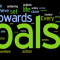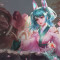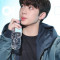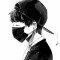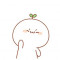## Tài trợ